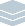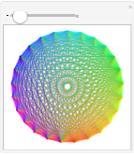Wolfram Archive
Wolfram Programming Lab is a legacy product.
All the same functionality and features, including access to Programming Lab Explorations, are available with Wolfram|One.
Start programming now. »
Try it now »
(no sign-in required)# Polygonal Mandalas

Make colorful mandala-like designs.

Run the code to draw a line through the 5 points of a pentagon (5-sided polygon). Try a different number of points:

CirclePoints gives points evenly spaced around a circle.

Draw the 5 corners of a pentagon (5-sided polygon):

Graphics[Point[CirclePoints]]

Connect the points with a line:

Graphics[Line[CirclePoints]]

The bottom of the pentagon is missing because the line is drawn from the first point to the last point, but not back to the first point again.

Graphics[Line[CirclePoints]]

Draw a line joining each pair of points. Try a different number of points:

This gives a list of every pair of numbers (2-tuple) drawn from the list {1,2,3}:

Tuples[{1, 2, 3}, 2]

This gives a list of every pair of points drawn from the list of three points evenly spaced around a circle:

Tuples[CirclePoints, 2]

Map (/@) applies a function, like Line, to every element of a list.

Draw a line connecting each pair of points:

Graphics[Line /@ Tuples[CirclePoints, 2]]

Graphics[Line /@ Tuples[CirclePoints, 2]]

Show pictures for successively more points. Try starting and ending numbers other than 4 and 20:

Table makes lists of things.

This makes a list of numbers from 4 to 20 in steps of 1:

Table[n, {n, 4, 20, 1}]

This makes a list of figures with 4 to 20 points:

Table[Graphics[Line /@ Tuples[CirclePoints[n], 2]], {n, 4, 20, 1}]

Table[Graphics[Line /@ Tuples[CirclePoints[n], 2]], {n, 4, 20}]

Make it interactive. Drag the slider to change the number of points:

Replace Table with Manipulate to get a slider that you can control the number of points with:

Manipulate[ Graphics[Line /@ Tuples[CirclePoints[n], 2]], {n, 4, 20, 1}]

Manipulate[ Graphics[Line /@ Tuples[CirclePoints[n], 2]], {n, 4, 20, 1}]

Make it colorful:

Were going to need a few new concepts to make a colored line figure. Lets take it in small steps.

A pure function is a function that doesnt have a name.

This pure function (indicated by # and &) adds 1 to a number:

(# + 1) &

Use the pure function with Map (/@) to add 1 to every number in a list:

(# + 1) & /@ {9, 3, 12}

An equivalent way to write the same thing is to use the function Map instead of the /@ shorthand:

Map[(# + 1) &, {9, 3, 12}]

MapIndexed is like Map. In fact, you get the same result if you use MapIndexed instead of Map:

MapIndexed[(# + 1) &, {9, 3, 12}]

But MapIndexed makes the list position of each element the function is applied to available to the function. Use #2 to get the position:

MapIndexed[{(# + 1), #2} &, {9, 3, 12}]

Use First to extract the position number from the list:

MapIndexed[{(# + 1), First[#2]} &, {9, 3, 12}]

Youll use the list position to specify the colors of lines in a line figure.

Hue gives different colors for different values between 0 and 1:

Table[Hue[h/10], {h, 0, 10, 1}]

You can use Hue to color objects in Graphics:

Graphics[{Hue[0.8], Line[{{0, 0}, {1, 1}}]}]

Now you have all the concepts you need to color the lines in a figure.

This is the basic figure with black lines:

Graphics[Map[Line, Tuples[CirclePoints, 2]]]

Use MapIndexed to color the lines according to their positions in the list. Dividing the Hue value by 12 gives 12 different colors of lines:

Graphics[MapIndexed[{Hue[First[#2]/12], Line[#]} &, Tuples[CirclePoints, 2]]]

Add color to the interactive figure:

Manipulate[ Graphics[MapIndexed[{Hue[First[#2]/n], Line[#]} &, Tuples[CirclePoints[n], 2]]], {n, 4, 30, 1}]

Manipulate[ Graphics[MapIndexed[{Hue[First[#2]/n], Line[#]} &, Tuples[CirclePoints[n], 2]]], {n, 4, 30, 1}]

Share Itmake a website with an interactive mandala:

Deploy the Manipulate to the Wolfram Cloud, where anyone with a browser can use it:

CloudDeploy[ Manipulate[ Graphics[MapIndexed[{Hue[First[#2]/n], Line[#]} &, Tuples[CirclePoints[n], 2]]], {n, 4, 30, 1}], Permissions -> "Public" ]

Click the link in the output to visit the site.